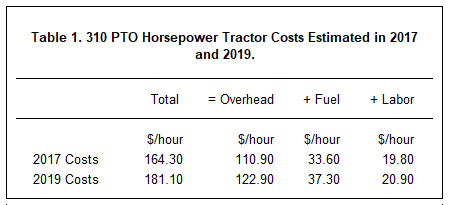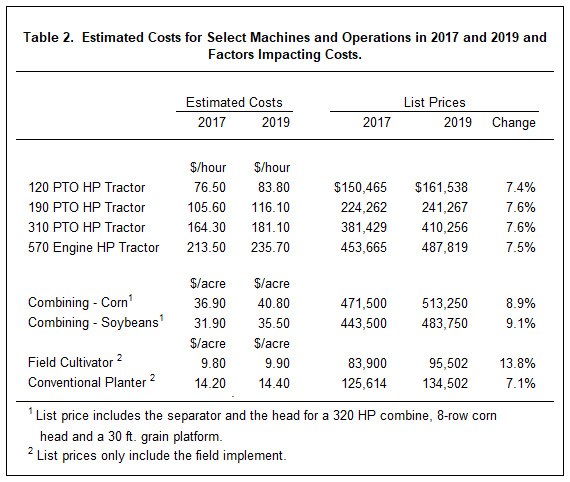# Machinery Cost Estimates for 2019

By: Dale Lattz and Gary Schnitkey, Department of Agricultural and Consumer Economics University of Illinois. farmdoc Daily

Machinery cost estimates for agricultural uses have been updated for 2019. The last time machinery costs were released on farmdoc was in 2017.  Between 2017 and 2019, most per acre costs increased between 7 and 9 percent.

Machinery Cost Estimates for 2019

Estimated machinery costs often are used to set custom rates when one individual performs a field operation for another individual.  We provide machinery cost estimates which may be useful in setting custom rates.  An additional amount for profit should be added to our cost estimates, as we have not added an amount for returns.

Machinery costs estimates are available in the management section of farmdoc in five publications:

1. Machinery Cost Estimates: Field Operations (https://farmdoc.illinois.edu/handbook/field-operations) – Shows per acre costs of performing field operations such as chisel plowing, field cultivating, planting, and spraying.
2. Machinery Cost Estimates: Tractors (https://farmdoc.illinois.edu/handbook/tractor-costs) – Shows per hour costs of operating various horsepower tractors.
3. Machinery Cost Estimates: Harvesting (https://farmdoc.illinois.edu/handbook/harvest-operations). Shows per acre costs of harvesting corn and soybeans, as well as use of grain cart.
4. Machinery Cost Estimates: Forage Field Operations (https://farmdoc.illinois.edu/handbook/forage-operations)– Shows per acre costs of performing forage operations.
5. Machinery Cost Estimates: Summary (https://farmdoc.illinois.edu/handbook/machinery-cost-estimates-summary) – Contains summary tables from the above operations.

Factors Impacting Estimated Machinery Costs

Costs are estimated in both 2017 and 2019 using an estimation approach that relies on formulas developed by the American Society of Agricultural Engineers (ASAE).  From 2017 to 2019, most of the estimated machinery costs went up.

Factors impacting costs are illustrated for a 310 PTO horsepower tractor with front wheel assist (per hour tractor costs are shown in the publication Machinery Costs Estimates: Tractor (https://farmdoc.illinois.edu/handbook/machinery-cost-estimates-summary).  Total costs were estimated at \$164.30 per hour in 2017 (see Table 1.  Per hour costs increased by \$16.80 per hour to \$181.10. per hour in 2019 (see Table 1).

Per hour costs are divided into overhead, fuel, and labor categories.  Overhead includes depreciation, interest, insurance and housing, and repairs.  Formulas are used to estimate each cost, and the purchase price enters into those formulas.  The purchase price is assumed to equal 85% of the list price of the tractor.  Between 2017 and 2019, the list price on a 310 HP tractor increased by by 7.6% from \$381,439 in 2017 to \$410,256 in 2019.  Overhead costs also increased because interest rates were increased from 5.0% in 2017 to 5.5% in 2019.  List price and interest rate increases resulted in an increased in overhead costs from \$110.90 in 2017 to \$122.90 in 2019 (see Table 1).  More detail on the approaches used to estimate overhead costs are given in the Machinery Cost Estimates: Tractors (https://farmdoc.illinois.edu/handbook/tractor-costs) publication.

Fuel use on a 310 horsepower tractor is estimated at 13.6 gallons per hour.  The diesel fuel price was increased from \$2.25 per gallon in 2017 to \$2.50 per gallon in 2019.  Due to the fuel price increase, fuel costs for the 310 horsepower tractor increased from \$33.60 per hour in 2017 to \$37.30 per hour in 2019 (see Table 1).

Labor costs per hour were increased from \$19.80 per hour in 2017 to \$20.90 per hour in 2019 (see Table 1).  An \$18 per hour rate was used in 2017 compared to \$19 per hour rate in 2019.  Labor time is assumed to be 1.1 times the tractor hour resulting in a 10% higher cost per tractor hour.

Table 2 shows estimated costs in 2017 and 2019 for several different tractors sizes, combining corn and soybeans, a field cultivator, and a conventional planter.  In all cases, list prices for the equipment went up and ranged from 7.1% to 13.8%.  Per acre costs did not necessarily go up as much depending on assumptions for field efficiency and acres covered.Factors Impacting Per Hour and Per Acre Costs

Per acre cost estimates are made based on assumptions concerning prices, interest rates, and use.  In calculating costs, for example, 300 hours of use were assumed for tractors.  Use has a large impact on per hour costs.  Higher hours of use will lower per hour costs as depreciation and interest costs are spread over more hours.  Similarly, more acres of use will generally reduce per acre costs of a field implement.Summary

Machinery cost estimates for 2019 have been released.  Costs for most operations are higher in 2019 than in 2017.  Most of the increases are due to increases in list prices of machines.  Fuel price and interest rate increases also contributed to higher prices.